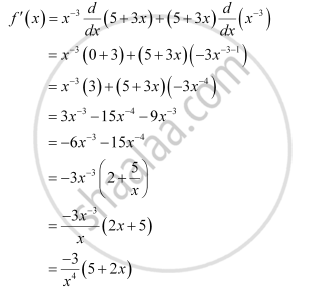CBSE (Arts) Class 11CBSE
Share

# Find the Derivative Of X–3 (5 + 3x) - CBSE (Arts) Class 11 - Mathematics

ConceptDerivative Algebra of Derivative of Functions

#### Question

Find the derivative of x–3 (5 + 3x)

#### Solution

Let f (x) = x– 3 (5 + 3x)

By Leibnitz product rule,Is there an error in this question or solution?

#### APPEARS IN

NCERT Solution for Mathematics Textbook for Class 11 (2018 to Current)
Chapter 13: Limits and Derivatives
Q: 9.3 | Page no. 313

#### Video TutorialsVIEW ALL 

Solution Find the Derivative Of X–3 (5 + 3x) Concept: Derivative - Algebra of Derivative of Functions.
S